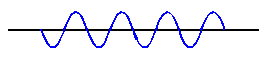# sine wave

(redirected from Frequency, periodicity, amplitude for sinusoids)
Also found in: Dictionary, Thesaurus, Medical, Financial.

## Sine wave

A wave having a form which, if plotted, would be the same as that of a trigonometric sine or cosine function. The sine wave may be thought of as the projection on a plane of the path of a point moving around a circle at uniform speed. It is characteristic of one-dimensional vibrations and one-dimensional waves having no dissipation. See Harmonic motion

The sine wave is the basic function employed in harmonic analysis. It can be shown that any complex motion in a one-dimensional system can be described as the superposition of sine waves having certain amplitude and phase relationships. The technique for determining these relationships is known as Fourier analysis. See Wave equation, Wave motion

McGraw-Hill Concise Encyclopedia of Physics. © 2002 by The McGraw-Hill Companies, Inc.

## sine wave

[′sīn ‚wāv]
(physics)
A wave whose amplitude varies as the sine of a linear function of time. Also known as sinusoidal wave.
McGraw-Hill Dictionary of Scientific & Technical Terms, 6E, Copyright © 2003 by The McGraw-Hill Companies, Inc.

## sine wave

A wave form containing only one frequency; the amplitude of the periodic oscillation is a sinusoidal function of time. Also see pure tone.
McGraw-Hill Dictionary of Architecture and Construction. Copyright © 2003 by McGraw-Hill Companies, Inc.

## sine wave

(mathematics)
A waveform of a single constant frequency and amplitude that continues for all time.

Compare wavelet.
A Sine Wave